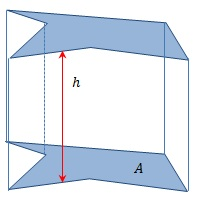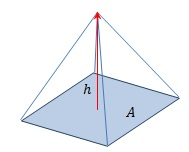# How to Find the Volume of Cube, Prism and Pyramid

Since cube , prism , and pyramid are three of the basic solid objects found in geometry, knowing how to find the volume of cube, prism and pyramid is essential. In mathematics and physical sciences and engineering, the properties of these objects have great importance. Most of the time the geometric and physical properties of a more complex object are always approximated using the properties of the solid objects. Volume is one such property.

## How to Find the Volume of a Cube

Cube is a solid object with six square faces that meet in right angles. It has 8 vertices and 12 edges and its edges are equal in length. The volume of the cube is the fundamental (perhaps the easiest volume to determine) of volume of all the solid objects. The volume of a cube is given by,

Vcube = a3, where a is length of its edges.## How to Find the Volume of a Prism

A prism is a polyhedron; it is a solid object consisting of two congruent (similar in shape and equal in size) polygonal faces with their identical edges connected by rectangles. The polygonal face is known as the base of the prism, and the two bases are parallel to each other. However, it is not necessary that they are exactly positioned above the other. If they are positioned exactly above each other, then the rectangular sides and the base meets at right angles. This kind of prism is known as a right angled prism.

If the area of the base (polygonal face) is A and the perpendicular height between the bases is h, then the volume of a prism is given by the formula,

Vprism = AhThe result holds true whether it is a right angle prism or not.

## How to Find the Volume of a Pyramid

The pyramid is also a polyhedron, with a polygonal base and a point (called the apex) connected by triangles extending from the edges. A pyramid has only one apex,but the number of vertices is dependent on polygonal base.

The volume of a pyramid with the base area A and perpendicular height to the apex h is given by,

Vpyramid = 1/3 Ah

## How to find the volume of a Cube, Prism and Pyramid – method

### Volume of a Cube

The cube is the easiest solid object to find the volume.

1. Find the length of one side (consider a)
2. Raise that value to the power of 3, i.e., a3 (find the cube)
3. The resulting value is the volume of the cube.

The unit of volume is the cube of the unit in which the length was measured.Therefore, if the sides were measured in meters, the volume is given in cubic meters.

### Volume of a Prism

1. Find the area of either base of the prism (A) and determine the perpendicular height between the two bases (h).
2. Product of the area h and the perpendicular height gives the volume of the prism.

Note: This result is valid for any type of prism, regular or non-regular.

### Volume of a Pyramid

1. Find the area of the base of the pyramid (A) and determine the perpendicular height from the base to the apex (h).
2. Take the product of the Area of the base and the perpendicular height. One third of the resulting values is the volume of the pyramid.

Note: This result is valid for any type of prism, regular or non-regular.

## How to find the volume of Cube, Prism and Pyramid – Examples

### Find the Volume of a Cube

1. An edge of a cube has a length 1.5m. Find the volume of the cube.

• The length of the cube is given as 1.5m. If not given directly, find the length using other geometric means or measuring.
• Take the third power of the length. That is (1.5)3=1.5×1.5×1.5=3.375m3
• A cube has a volume of 3.375 cubic meters.

### Find the Volume of a Prism

2. A triangular prism has a length of 20cm. The base of the prism is an isosceles triangle with equal sides forming an angle of 600. If the length of the side opposing the angle is 4cm, find the volume of the pyramid.

• First, determine the area of the base.By trigonometric ratios, we can determine the perpendicular height of the base triangle from the 4cm edge to the opposing vertex as 2 tan 600 =2×√3≅3.4641 cm. Therefore, the area of the base is1/2×4×3.4641=6.9298cm2
• The perpendicular height is given (as the length) as 20cm. Now, we can calculate the volume by multiplying the area of the base by the perpendicular height, such as Vprism=A×h=6.9298cm2×20cm=138.596cm3
• The volume of the pyramid is 138.596cm3.

### Find the Volume of a Pyramid

3. A rectangular right pyramid has a base with 40m in width and 60m in length. If the height to the apex of the pyramid from the base is 20m, find the volume enclosed by the surface of the pyramid.

• The area of the base can be simply determined by taking the product of the lengths of the two sides. Therefore, the area of the base is 40m×60m=2400m2
• The perpendicular height is given as 20m. Therefore, the volume of the pyramid is Vpyramid=1/3×2400m2×20m=16,000m3